Preliminaries Next: Constructing the Octonions Up: Introduction Previous: Introduction

## 1.1 Preliminaries

Before our tour begins, let us settle on some definitions. For us a vector space will always be a finite-dimensional module over the field of real numbers. An algebrawill be a vector space that is equipped with a bilinear map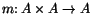called 'multiplication' and a nonzero element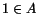called the 'unit' such that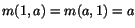. As usual, we abbreviate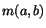as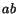. We do not assume our algebras are associative! Given an algebra, we will freely think of real numbers as elements of this algebra via the map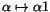.

An algebrais a division algebra if given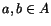with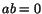, then eitheror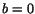. Equivalently,is a division algebra if the operations of left and right multiplication by any nonzero element are invertible. A normed division algebra is an algebrathat is also a normed vector space with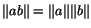. This implies thatis a division algebra and that.

We should warn the reader of some subtleties. We say an algebrahas multiplicative inverses if for any nonzero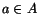there is an elementwith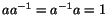. An associative algebra has multiplicative inverses iff it is a division algebra. However, this fails for nonassociative algebras! In Section 2.2 we shall construct algebras that have multiplicative inverses, but are not division algebras. On the other hand, we can construct a division algebra without multiplicative inverses by taking the quaternions and modifying the product slightly, setting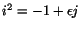for some small nonzero real number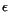while leaving the rest of the multiplication table unchanged. The element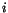then has both right and left inverses, but they are not equal. (We thank David Rusin for this example.)

There are three levels of associativity. An algebra is power-associative if the subalgebra generated by any one element is associative. It is alternative if the subalgebra generated by any two elements is associative. Finally, if the subalgebra generated by any three elements is associative, the algebra is associative.

As we shall see, the octonions are not associative, but they are alternative. How does one check a thing like this? By a theorem of Emil Artin , an algebrais alternative iff for allwe haveIn fact, any two of these equations implies the remaining one, so people usually take the first and last as the definition of 'alternative'. To see this fact, note that any algebra has a trilinear map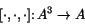called the associator, given byThe associator measures the failure of associativity just as the commutator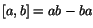measures the failure of commutativity. Now, the commutator is an alternating bilinear map, meaning that it switches sign whenever the two arguments are exchanged: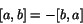or equivalently, that it vanishes when they are equal: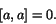This raises the question of whether the associator is alternating too. In fact, this holds precisely whenis alternative! The reason is that each equation in (1.1) says that the associator vanishes when a certain pair of arguments are equal, or equivalently, that it changes sign when that pair of arguments is switched. Note, however, that if the associator changes sign when we switch theth and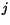th arguments, and also when we switch theth and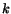th arguments, it must change sign when we switch theth andth. Thus any two of equations (1.1) imply the third.

Now we can say what is so great about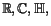and:

Theorem 1.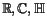, andare the only normed division algebras.

Theorem 2., andare the only alternative division algebras.

The first theorem goes back to an 1898 paper by Hurwitz . It was subsequently generalized in many directions, for example, to algebras over other fields. A version of the second theorem appears in an 1930 paper by Zorn  -- the guy with the lemma. For modern proofs of both these theorems, see Schafer's excellent book on nonassociative algebras . We sketch a couple proofs of Hurwitz's theorem in Section 2.3.

Note that we did not state thatandare the only division algebras. This is not true. For example, we have already described a way to get 4-dimensional division algebras that do not have multiplicative inverses. However, we do have this fact:

Theorem 3.   All division algebras have dimension 1, 2, 4, or 8.

This was independently proved by Kervaire  and Bott-Milnor  in 1958. We will say a bit about the proof in Section 3.1. However, in what follows our main focus will not be on general results about division algebras. Instead, we concentrate on special features of the octonions. Let us begin by constructing them.

Next: Constructing the Octonions Up: Introduction Previous: Introduction

© 2001 John Baez